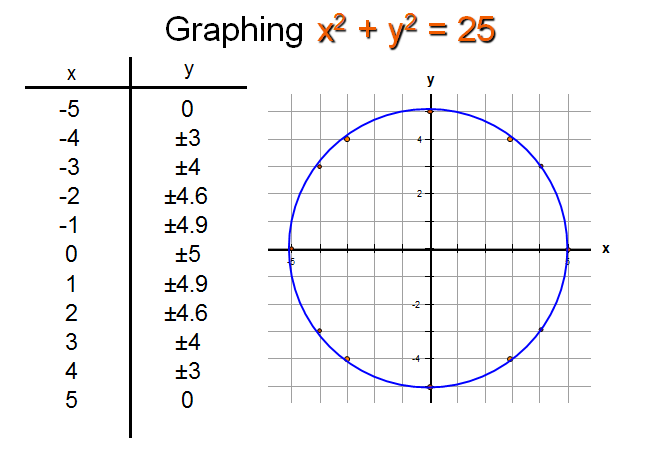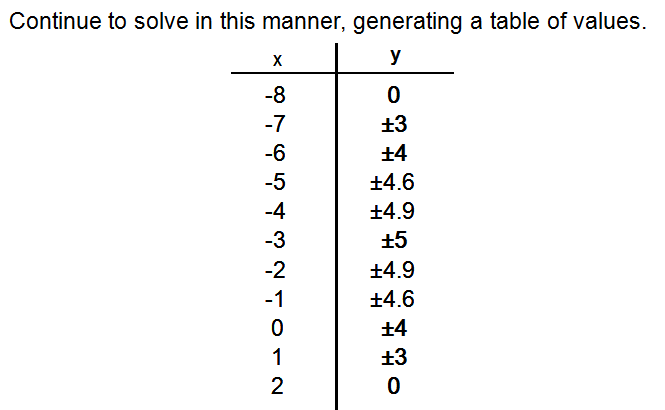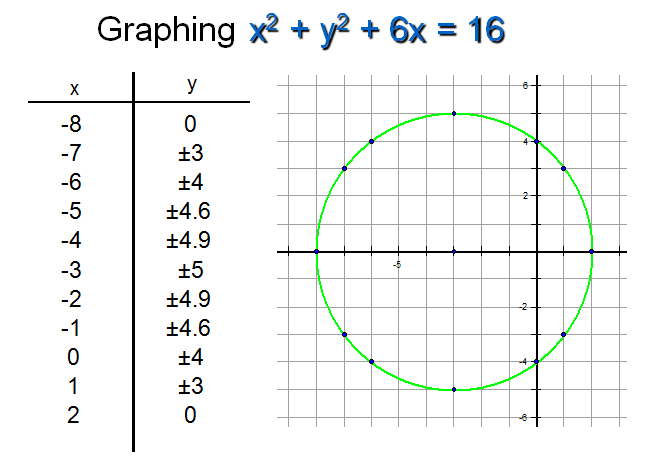# SCC Education

## Introduction of Conic section ,circle ,ellipse

in the form of y = Ax2 + Dx + F
where A, D, and F stand for constants, and A ≠ 0
This quadratic relation is a parabola, and it is the only one that can be a function.It does not have to be a function, though.A parabola is determined by a plane intersecting a cone and is therefore considered a conic sectionSeries-formulas
The-binomial-theorem
Analytic-trigonometry-double-angle-half angle
solved-problems-on-limits-and-continuity
geometric-progressions
Basic-point-formulas-distance-midpoint
Two-point-form-normal-form parametric
limit-questions
set-relation-function-part1
permutation-combination
straight-lines
Three-diamensional-geometry
Trignometry

hyperbola